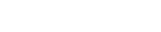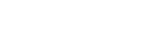# MATHEMATICS PAPER 1 - 2019 LAINAKU JOINT MOCK EVALUATION EXAMINATION

SECTION 1 (50 MARKS)
Answer all questions in this section in the spaces provided.

1. Without using a calculator or mathematical table evaluate

1/3 of (2 ¾ - 5 ½) x 3 6/7 ÷ 9/4                                                               (3mks)

1. Solve for x in the equation. (3mks)1. A line P whose equation is y =1/3x+4  is parallel to another line Q. Find the equation of line Q in the form y= mx + c given that it passes through Point (3, 6) (3mks)
2. Using reciprocals, cubes and squire tables, evaluate correct to 4 significant figures: (4mks 1.897 23. A point P (2, 3) is mapped onto P’ (-7, 0) under an enlargement with scale factor of -2 without drawing find the centre of enlargement.                                                             (3mks)
4. A businessman bought 100 textbooks and 80 pens for sh. 25,600. If she had bought twice as many textbooks and half as many pens she would have paid sh. 7,400 less. Find the cost of one textbook and one pen. (3 mks)
5. The table below shows the number of faulty balls from 40 samples.
 No. of faulty balls (c) 0 1 2 3 4 5 Frequency 20 8 6 1 1 4

Calculate the mean.
1. Draw a sketch and find the area in hectares of a coffee field whose measurements are entered in a field book as shown below. Take XY = 200m as the baseline. (4 marks)
 Y 180 40 to Q To R 80 140 To S 160 100 40 100 to P X
1. In the following figure, 0 is the centre of the circle. Given that Angle ABC 60°, find the value of the angle ADC. (2mks)2. From the top of a cliff the angle of depression of a ship when it is at A is 30o. When the ship moves 100m to point B, nearer the cliff, the angle of depression becomes 45o. Find the height of the cliff leaving your answer to 3.s.f(4mks)
3. During a football match, sh. 1,462,800was realized from stadium entrance fees. If the entrance fee was sh. 80 per person, calculate how many fans paid to watch match. (2 mks)
4. A Kenya Bank buys and sells foreign currencies as shown
1 Euro                        84.15                                     84.26
100 Japanese Yen       65.37                                      65.45
A Japanese travelling from France to Kenya had 5000 Euros. He converted all the 5000 Euros to Kenya shillings at the bank. While in Kenya, he spent a total of Kshs. 289850 and then converted the remaining Kenya shillings to Japanese Yens at the Bank. Calculate the amount in Japanese Yen that he received.(3mks)
5. The width of a rectangular hall of Ruiri Girls Secondary School is 16m less than its length. Calculate the length of the hall if its area is 132m2. Hence calculate its perimeter. (4 mks)
6. The volume of a hemisphere is 41.2cm3.Calculate, correct to one decimal place, the radius of the hemisphere    (3mks)
7. The figure below shows a triangle ABC in which AB = 6cm, BC = 11cm and angle ABC = 1100. Calculate to the decimal places the length of AC.           (3mks)8. A regular polygon has internal angle of 1500 and side of length 10cm.
1. Find the number of sides of the polygon.                               (2mks)
2. Find the perimeter of the polygon.                                         (2mks)

SECTION 1I (50 MARKS)
Answer any five questions from this Section.

9. A matatu left town K at 7.00a.m and travelled towards town M at an average speed of 60km/hr.A car left town M at 9.00a.m and travelled towards K at an average speed of 80km/hr. The distance between the two towns is 324km.Find:-
1. The time each vehicle arrived at their destination
1. Matatu                                                                                            (2mks)
2. Car                                                                                     (2mks)
1. the distance the matatuhad covered before the car started to move from town M to town K
2. The time the two vehicles met on the way                                    (3mks)
3. How far the car was from town K when they met                        (2mks)
1. The figure below shows a sector of a circle. If the radius OA = 14cm and the angle AOB = 720.1. Calculate the area of the sector.                                                                             (2mks
2. The sector is folded to form a cone. Calculate:-
1. The radius of the cone formed.                                                                  (2mks)
2. The volume of the solid formed.                                                                (3mks)
3. A solid cone of same size as the one in (b) above is melted down and casted into circular washers. Each washer has an external diameter of 4cm, internal diameter of 1½ cm and 0.3cm thick. Calculate number of washers made.                                    (3mks)
1. Find the inverse ofmatrix A = (2mks)
2. Okelo bought 5 physics   book and six mathematics book for a total of Ksh.2440.Ali bought 7 physics book and 9 mathematics books for a total   cost   of ksh.3560.
1. Form a matrix equation to represent the a book   information   (1mk)
2. Use matrix method to find the price of a physics book and that of a mathematic (3mks
3. A school bought 36physics books and 50mathematics books. Adiscount of 5% wasallowed oneach Physics book whereas adiscount of 8 % was allowed on each Mathematics book. Calculate the percentagediscount onthe cost of all the books bought. (4mks)
2. Forty students in a form 2 class were weighed and their masses recorded to the nearest kilogram as shown below.
45        48      56         39        47        36        45        37      46       35         43       51     42
47        47      40         46        41        45        43        46      54        42        51       39     42
45        44     49          50          46       39       42        48       50       38          45      35    52        46
1. Starting with the class 35-39 tabulate this data in a frequency table           (3mks)
2. Find the modal class                                                 (1mk)
3. Calculate the mean mass of the students                                                      (3mks)
4. Estimate the median mass                                                                               (3mks)
1. In triangle OAB, OA = a and OB = b. Points P and T divide OB and AB in the ratio 2:3 and 1:3 respectively. Lines OT and AP intersect at Q.
1. Draw the diagram to represent the above information.                                  (1mk)
2. Express OP and AP in term of a and b .                                                         (2mks
3. Express OT in terms of a and b .                                                        (1mk)
4. Given further that OQ = tOT and AQ = sAP, express OQ in two ways and hence find the values of s and t.                                                               (6mks)
1. A metal R is an alloy of two metals X and Y. Metal X has a mass of 70g and a density of 16g/cm3. Metal Y has a mass of 19g and a density of 4g/cm3.
1. Calculate the density of the metal R.                                (4mks)
2. If metal R is divided into two equal parts and each half reinforced by adding metal X to get to initial volume. Find the density of the new alloy.                (4mks)
3. The two metals are mixed in a ratio of 4:1 respectively. What is the density of the alloy?(2mks)
1. Meshach and Kelvin contributed shs. 60,000 and sh. 90,000 respectively in order to start business. They employed a manager and agreed to pay him sh. 4,500 per month from the profit made each year. They also agreed that 20% of the profit made each year would be put back into the business while the rest would be shared between them in the ratio of their initial contribution. During the first year they made a profit of sh. 365,000. Calculate:-
1. The manager’s annual salary for that year              (1mk)
2. The money put back into business that year.                      (2mks)
3. The business net profit for that year.                                  (2mks)
4. How much each partner received that year. (3mks)
5. The capital for the following year.                         (2mks)
1. A car starts from rest and builds up a speed of 40m/s in 1 min 40 seconds. It then travels at this speed for 5 minutes. Brakes are then applied and the car is brought to a halt in 2 minutes.
1. Draw a velocity-time graph to represent the information above.                          (3mks)2. Use your graph to find
1. The initial acceleration.                                                                       (2mks)
2. The deceleration when the car is brought to a halt.                            (2mks)
3. Thedistance traveled in km.                                                                     (3mks)## MARKING SCHEME#### Download MATHEMATICS PAPER 1 - 2019 LAINAKU JOINT MOCK EVALUATION EXAMINATION.

• ✔ To read offline at any time.
• ✔ To Print at your convenience
• ✔ Share Easily with Friends / Students